# MA311exam 1999 pdfLoading previews...
 tensorial notation, Eulerian and Lagrangian flow and Navier-Stokes equations Text Download (32kB)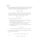tensorial notation, Eulerian and Lagrangian flow and Navier-Stokes equations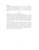viscous flow, velocity fields and the Navier-Stokes equations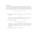flow velocity and mass flow rate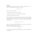stream function, Reynolds number and boundary-layer equations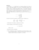Reynolds number and Stokes stream function
6 files in this resource

## MA311exam 1999 pdf

Exam questions and solutions in PDFView Item